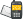It is currently 24 May 2020, 19:59### GMAT Club Daily Prep

#### Thank you for using the timer - this advanced tool can estimate your performance and suggest more practice questions. We have subscribed you to Daily Prep Questions via email.

Customized
for You

we will pick new questions that match your level based on your Timer History

Track

every week, we’ll send you an estimated GMAT score based on your performance

Practice
Pays

we will pick new questions that match your level based on your Timer History

#### Not interested in getting valuable practice questions and articles delivered to your email? No problem, unsubscribe here.# If Band D are centers of the circles shown in the figure a bQuestion banks Downloads My Bookmarks Reviews Important topics
Author Message
TAGS:
FounderJoined: 18 Apr 2015
Posts: 11096
Followers: 237

Kudos [?]: 2772 , given: 10521

If Band D are centers of the circles shown in the figure a b [#permalink]
Expert's post00:00

Question Stats:40% (02:21) correct60% (05:11) wrongbased on 5 sessions
Attachment:#greprepclub If Band D are centers of the circles shown.jpg [ 22.12 KiB | Viewed 148 times ]

If $$B$$ and $$D$$ are centers of the circles shown in the figure above and if $$BD = 12$$, $$BC = 2$$, and $$DE= 3$$, then $$AB =$$

(A) 12

(B) 14

(C) 21

(D) 22

(E) 24
[Reveal] Spoiler: OA

_________________

Need Practice? 20 Free GRE Quant Tests available for free with 20 Kudos
GRE Prep Club Members of the Month: Each member of the month will get three months free access of GRE Prep Club tests.InternJoined: 17 Feb 2020
Posts: 44
Followers: 1

Kudos [?]: 32  , given: 4

Re: If Band D are centers of the circles shown in the figure a b [#permalink]
1
KUDOS
Hey, Carcass Be=2, is it correct?InternJoined: 17 Feb 2020
Posts: 44
Followers: 1

Kudos [?]: 32  , given: 4

Re: If Band D are centers of the circles shown in the figure a b [#permalink]
1
KUDOS
I think it's not Be but BC, am I right?
FounderJoined: 18 Apr 2015
Posts: 11096
Followers: 237

Kudos [?]: 2772 , given: 10521

Re: If Band D are centers of the circles shown in the figure a b [#permalink]
Expert's post
Yes Sir. Fixed. Of course was BC.

When you take the big book stems sometimes this happens because the book in pdf is really old.

Thank you so much. I am sorry of the typo.

Resrds
_________________

Need Practice? 20 Free GRE Quant Tests available for free with 20 Kudos
GRE Prep Club Members of the Month: Each member of the month will get three months free access of GRE Prep Club tests.

InternJoined: 13 Mar 2020
Posts: 17
Followers: 1

Kudos [?]: 3 , given: 10

Re: If Band D are centers of the circles shown in the figure a b [#permalink]
which property is used hereInternJoined: 17 Feb 2020
Posts: 44
Followers: 1

Kudos [?]: 32  , given: 4

Re: If Band D are centers of the circles shown in the figure a b [#permalink]
1
KUDOS
triangle similarity or congruent triangles
InternJoined: 13 Mar 2020
Posts: 17
Followers: 1

Kudos [?]: 3 , given: 10

Re: If Band D are centers of the circles shown in the figure a b [#permalink]
thank u so much

Posted from my mobile deviceFounderJoined: 18 Apr 2015
Posts: 11096
Followers: 237

Kudos [?]: 2772 , given: 10521

Re: If Band D are centers of the circles shown in the figure a b [#permalink]
Expert's post
We have to use properties of similar triangle here.

Triangle ACB is similar to AED
$$\frac{AD}{AB} = \frac{DE}{BC}$$ => $$\frac{x}{x}+12 = \frac{3}{2}$$ => 2x+24 = 3x => x=24

Orrrr

We know Diameter of smaller circle = 4 and Diameter of larger circle = 6
Hence
$$\frac{AB}{AD} = \frac{4}{6}$$

$$\frac{x}{x}+12 = \frac{4}{6}$$
x= 24
E
_________________

Need Practice? 20 Free GRE Quant Tests available for free with 20 Kudos
GRE Prep Club Members of the Month: Each member of the month will get three months free access of GRE Prep Club tests.

InternJoined: 24 Jan 2020
Posts: 19
Followers: 0

Kudos [?]: 16 , given: 0

Re: If Band D are centers of the circles shown in the figure a b [#permalink]
Triangle ABC is similar to ADE hence

$$\frac{AB}{AD}$$ = $$\frac{BC}{DE}$$

Lets value of AB = x

Hence $$\frac{x}{(AB + BD)}$$ = $$\frac{2}{3}$$

$$\frac{x}{(x+12)}$$ = $$\frac{2}{3}$$

3x = 2x + 24

x=24Re: If Band D are centers of the circles shown in the figure a b   [#permalink] 23 May 2020, 04:31
Display posts from previous: Sort by

# If Band D are centers of the circles shown in the figure a bQuestion banks Downloads My Bookmarks Reviews Important topicsPowered by phpBB © phpBB Group Kindly note that the GRE® test is a registered trademark of the Educational Testing Service®, and this site has neither been reviewed nor endorsed by ETS®.